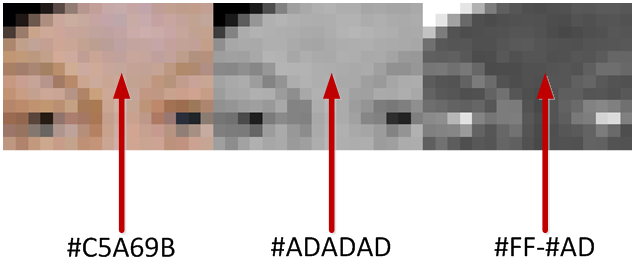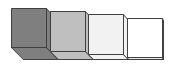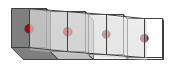# So, how do you convert an image to a Lithophane?

In short, you take your image, convert it to monochrome, invert it and then use the brightness of each pixel as the height of the output.

To elaborate:Load a coloured image, convert the brightness of each pixel to monochrome and then use its negative.If we look at a pixel in the middle of Henry VIII’s forehead, we can see that the pixel has the values of C5 for Red, A6 for Green and 9B for Blue that is 76% of full brightness on the red channel,68% on the green channel and 60% on the blue.

To convert this we could average the three values and put that value into each channel, but the human eye sees each of the colours slightly differently, green appearing much brighter than blue for instance. In fact we see brightness in approximately the proportions R=30%,G=59%,B=11% so multiplying the colour channels by the 0.3,0.59 and 0.11 respectively and adding them together gives us a much more realistic monochrome image.

You’ll note in the second image, the red,green and blue channels are now all the same and we have effectively reduced the image from 24 to 8 bits in resolution, this will help save some space and speed up processing.

As we are going to be passing light through our image, we need the lithophane to be more opaque where the image is darker and more transparent where the image is lighter. i.e. thicker = darker, thinner = lighter. The final stage of 2D processing is to invert each pixel i.e. where it is white (Highest value = 255) we need thin plastic and where it is black (Lowest value = 0) we need thick plastic. As each channel is the same , we can use one channel’s value, subtracting each brightness from 255 gives us a level from 0-255 in the same form as needed for the output. We can simply multiply the resultant value by a scale to set the maximum lithophane thickness and add an offset for the minimum lithophane thickness, for example:

`thickness = (inverse_pixel_brightness * 0.02mm) + 0.2mm`

Will give us a lithophane that is 0.2mm thick at its thinnest point and 12.9mm thick at its thickest.

We can now directly translate those pixel values into 3 dimensional coordinates for our lithophane, we have two options regarding how our lithophane is structured, we can use a cube to represent each pixel, and set the Z-height of the cube based on the inverse brightness value for each X/Y pixel coordinate:Or, we can create a surface with smooth transitions between the levels of each pixel:Which version you use will depend on your image and how “rough” it is, although the smooth version looks nicest, you may find that there are lots of small high or low features they become very small spikes and do not print well.  On the other hand, the array of cube pixel towers may take a long tome to slice. We will develop both of these methods and see how the look in practice.

So lets start by writing the code for the 2D image processing.

```<body>
<img src="mountain.jpg" onclick="onImageClicked(event);">
<canvas id="outputcanvas" style="width:320px;height:240px""> </canvas>
</body>```
```function onImageClicked(event) {
var image = event.target; // the image that was clicked

// point at canvas element that will show image data
// once we've processed it
var canvas = document.getElementById("outputcanvas");
// make our canvas the same size as the image
canvas.width = image.naturalWidth;
canvas.height = image.naturalHeight;

// we'll need the 2D context to manipulate the data
var canvas_context = canvas.getContext("2d");
canvas_context.drawImage(image, 0, 0); // draw the image on our canvas

// image_data points to the image metadata including each pixel value
var image_data = canvas_context.getImageData(0, 0,
image.naturalWidth, image.naturalHeight);
// pixels points to the canvas pixel array, arranged in 4 byte
// blocks of Red, Green, Blue and Alpha channel
var pixels = image_data.data;

var numb_pixels=pixels.length/4; // the number of pixels to process

// an array to hold the result data
var height_data = new Uint8Array(numb_pixels);

var image_pixel_offset=0;// current image pixel being processed
// go through each pixel in the image
for (var height_pixel_index = 0;
height_pixel_index < numb_pixels;
height_pixel_index++) {

// extract red,green and blue from pixel array
var red_channel = pixels[image_pixel_offset ],
green_channel = pixels[image_pixel_offset + 1],
blue_channel = pixels[image_pixel_offset + 2];

// create negative monochrome value from red, green and blue values
var negative_average = 255 - (red_channel * 0.299 +
green_channel * 0.587 +
blue_channel * 0.114);

// store value in height array
height_data[height_pixel_index]=negative_average;

// store value back in canvas for display of negative monochrome image
pixels[image_pixel_offset] =
pixels[image_pixel_offset + 1] =
pixels[image_pixel_offset + 2] =
negative_average;

image_pixel_offset+=4; // offest of next pixel in RGBA byte array
}

// display modified image
canvas_context.putImageData(image_data, 0, 0, 0, 0,
image_data.width, image_data.height);

// create 3D lithophane using height data
setLevels(height_data, image_data.width, image_data.height);
}
function setLevels(heightData, width, height) {
// TODO - create 3D data from height data
}```